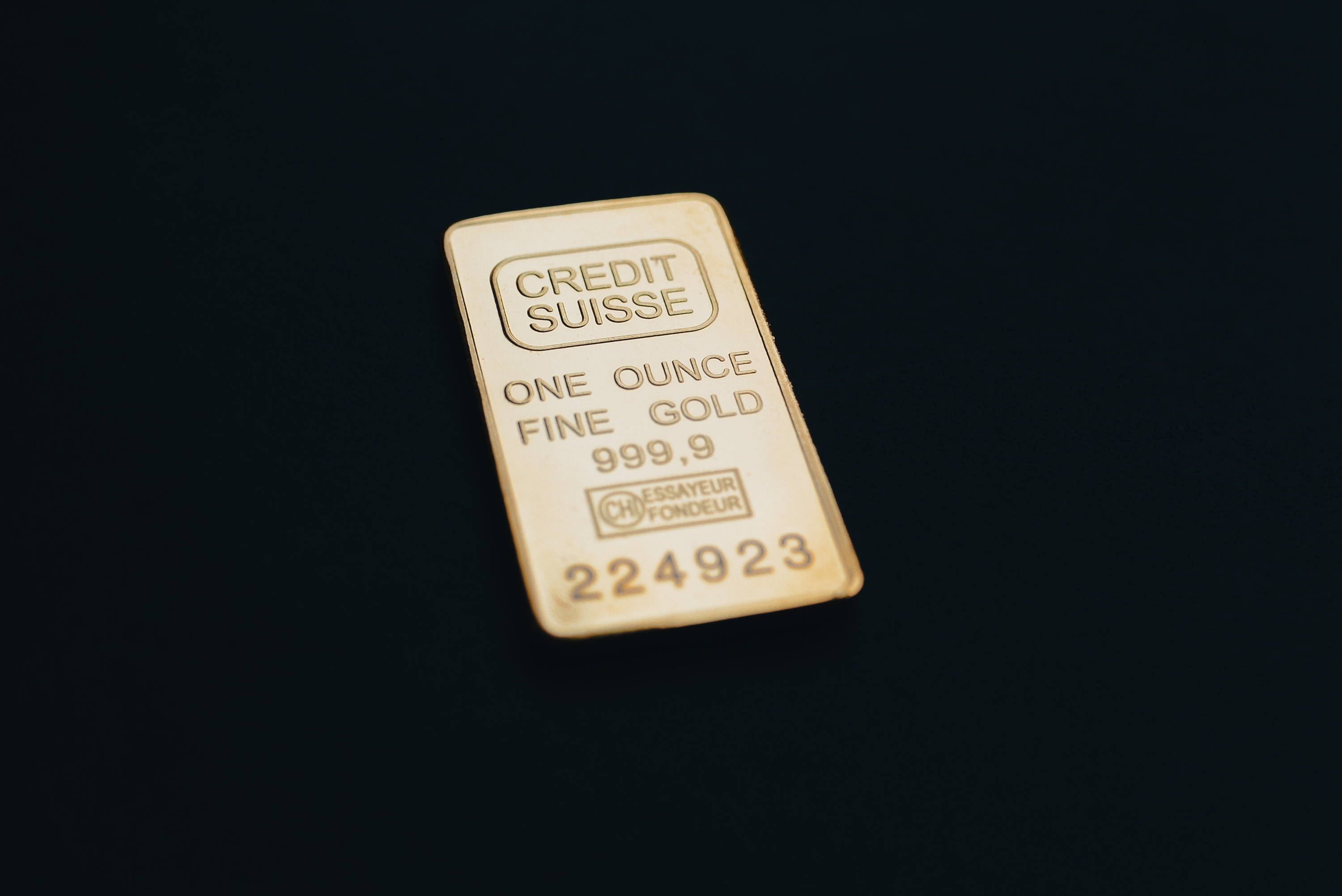Konnichi wa / Great Outdoors

## Introduction

When it comes to precise measurements, it is important to understand how many ounces are in a pound.

Whether you are measuring out ingredients for a recipe, calculating shipping costs, or dealing with commodities in the market, having an accurate understanding of ounces and pounds is key.

This article will explain the different types of ounces and how many ounces are in a pound.

Watch this video on how many ounces are in a pound!

## Etymology

The word "ounce" comes from the Latin uncia, which means "one-twelfth part."

This is because the ounce was originally one-twelfth of a Roman pound.

The abbreviation "oz" is derived from the Italian onza, which is also derived from the Latin uncia.

## Definitions

The ounce is a unit of measurement for mass, weight, and volume.

It is used in many different contexts, such as to measure food portions, postal items, areal density of fabric and paper, and boxing gloves.

## Currently in Use

There are two types of ounces currently in use: the international avoirdupois ounce and the international troy ounce.

## International Avoirdupois Ounce

The international avoirdupois ounce (abbreviated oz) is defined as exactly 28.349523125 grams.

This is the unit of measurement used in the United States and Commonwealth countries to measure packaged foods and food portions, postal items, areal density of fabric and paper, boxing gloves, and so on.

## International Troy Ounce

The international troy ounce (abbreviated oz t) is equal to 480 grains and is equal to exactly 31.1034768 grams.

It is primarily used to express the mass of precious metals such as gold, silver, platinum, palladium, and rhodium in the commodities market.

There are 12 troy ounces in a troy pound.

## How Many Ounces Are in a Pound?

In the avoirdupois system, sixteen ounces make up an avoirdupois pound, and the avoirdupois pound is defined as 7000 grains.

Therefore, there are 16 ounces in a pound.

## Measuring Precisely

When it comes to precise measurements, a digital scale is the most accurate way to measure out ingredients.

However, for smaller weight measurements, a measuring cup or spoon is often used.

For measuring dry ingredients, a dry ounce is equal to 28.35 grams, and for measuring liquid ingredients, a fluid ounce is equal to 29.57 grams.

## Different Units of Measurement

In the United States, the conventional ounce is the avoirdupois ounce, which is equal to 28.35 grams.

However, in the British Imperial system, the troy ounce is used, which is equal to 31.10 grams.

The metric system uses the mass m, which is equal to 1 gram.

## Conversions

When converting ounces to other units of measurement, it is important to remember that there are different types of ounces.

For example, a quarter ounce is equal to 7.08 grams in the avoirdupois system, but it is equal to 8.75 grams in the troy system.

## Rough Calculations

For a rough calculation, one can use a pound conversion chart to convert ounces to pounds. For example, one pound is equal to 16 ounces, so one can simply multiply the number of ounces by 16 to get the equivalent weight in pounds.

## Visual Learners

For visual learners, a pound conversion chart ounces conversion chart can be helpful in understanding how many ounces are in a pound.

Alternatively, if doing a simple conversion, one can use a measuring cup or spoon to measure out ingredients, or use a drip coffee maker to measure out a precise amount of liquid.

## Conclusion

Understanding how many ounces are in a pound is essential for accurate measurement of ingredients, calculating shipping costs, and more.

There are 16 ounces in a pound, and the two types of ounces currently in use are the international avoirdupois ounce and the international troy ounce.

For precise measurements, a digital scale is the most accurate way to measure out ingredients.

For smaller weight measurements, a measuring cup or spoon is often used.

When converting ounces to other units of measurement, it is important to remember that there are different types of ounces.

For a rough calculation, one can use a pound conversion chart to convert ounces to pounds.

For visual learners, a pound conversion chart can be helpful in understanding how many ounces are in a pound.

Looking for more on How Many Oz in a Pound and other topics? Try these:

“The mountains are calling and I must go.”

— John Muir